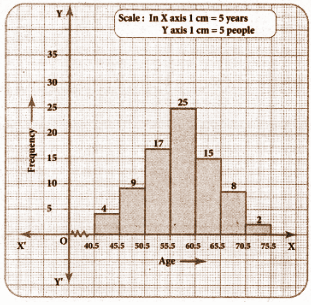# Samacheer Kalvi 8th Maths Book Solutions Term 3 Chapter 4 | Statistics Answers For Tamil Nadu Board

Samacheer Kalvi 8th Maths Book Solutions Term 3 Chapter 4 – Statistics is available here. The Samacheer Kalvi 8th Maths book answers of Term 3 Chapter 4, available at BYJU’S, contain step by step explanations designed by our Mathematics experts. All these important questions are based on the new pattern prescribed by the Tamil Nadu board. Students can also get the solutions of other chapters on Samacheer Kalvi 8th Maths solutions. Referring to the solutions after answering these Samacheer Kalvi Class 8 Maths Textbook Term 3 Chapter 4 questions is the best way to gauge the performance and their exam preparation level.

Term 3 Chapter 4 of the Samacheer Kalvi 8th Maths guide will help the students to solve problems related to data, types of data, frequency distribution, graphical representation of grouped and ungrouped data.

### Samacheer Kalvi 8th Maths Term 3 Chapter 4: Statistics Book Exercise 4.1 Questions and Solutions

Question 1: Represent the following data in an ungrouped frequency table which gives the number of children in 25 families. 1, 3, 0, 2, 5, 2, 3, 4, 1, 0, 5, 4, 3, 1, 3, 2, 5, 2, 1, 1, 2, 6, 2, 1, 4.

Solution:

The given data is raw in nature.

Ascending order 0, 1, 2, 3, 4, 5, 6.

 Number of children Tally marks Frequency 0 || 2 1 |||| | 6 2 |||| | 6 3 |||| 4 4 ||| 3 5 ||| 3 6 | 1 Total 25

Question 2: Draw a pie chart for the following information.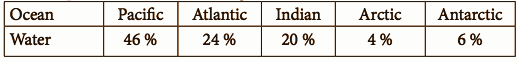Solution:

 Ocean Water (in %) Central angle Pacific 46 (46 / 100) * (360o) = 165.6o Atlantic 24 (24 / 100) * (360o) = 86.4o Indian 20 (20 / 100) * (360o) = 72o Arctic 4 (4 / 100) * (360o) = 14.4o Antarctic 6 (6 / 100) * (360o) = 21.6o Total 100 360o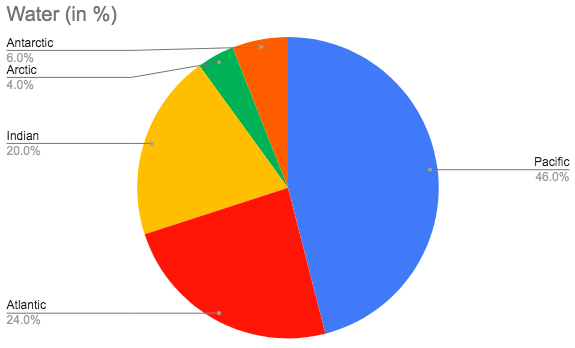Question 3: Write any five points from the given pie chart information regarding pollutants entering the oceans.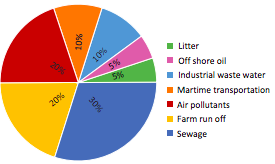Solution:

1] The pie chart gives information about the number of pollutants entering the ocean from various sources.

2] 30% of the ocean is polluted by sewage.

3] 20% of the ocean is polluted by air.

4] Farm runoff equally contributes as much as air in polluting ocean.

5] The observation is about offshore oil, litter, industrial wastewater, maritime transportation, air pollutants, farm runoff and sewage.

### Samacheer Kalvi 8th Maths Term 3 Chapter 4: Statistics Book Exercise 4.2 Questions and Solutions

Question 1: In a village, there are 570 people who have cell phones. An NGO surveys their cell phone usage. Based on this survey a histogram is drawn. Answer the following questions.

(i) How many people use the cell phone for less than 3 hours?

(ii) How many of them use the cell phone for more than 5 hours?

(iii) Are people using the cell phone for less than 1 hour?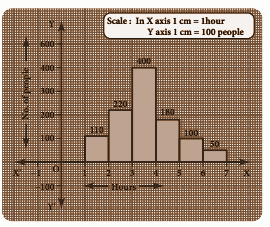Solution:

(i) 110 + 220 = 330 people use the cell phone for less than 3 hours.

(ii) 100 + 50 = 150 of them use the cell phone for more than 5 hours.

(iii) No

Question 2: Draw a histogram for the following data.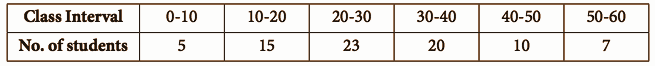Solution: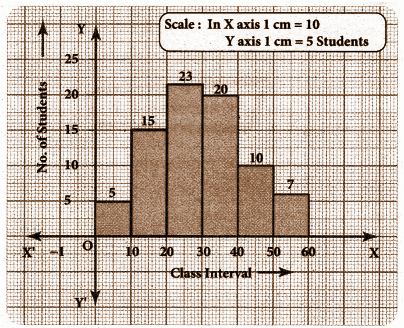Question 3: In a study of dental problem, the following data were obtained.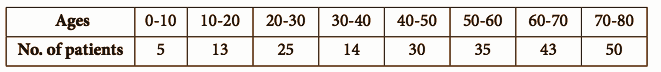Represent the above data by a frequency polygon.

Solution:

On finding the midpoints of the class interval,

 Ages Midpoint (x) Number of patients 0 – 10 5 5 10 – 20 15 13 20 – 30 25 25 30 – 40 35 14 40 – 50 45 30 50 – 60 55 35 60 – 70 65 43 70 – 80 75 50

A frequency polygon is plotted by taking midpoints and number of patients.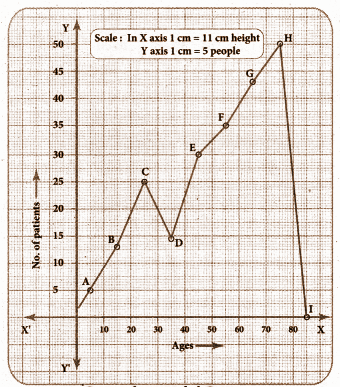### Samacheer Kalvi 8th Maths Term 3 Chapter 4: Statistics Book Exercise 4.3 Questions and Solutions

Question 1: Draw a pie chart for the given table.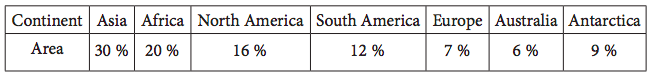Solution:

 Continent Area (in %) Central angle Asia 30 (30 / 100) * (360o) = 108o Africa 20 (20 / 100) * (360o) = 72o North America 16 (16 / 100) * (360o) = 57.6o South America 12 (12 / 100) * (360o) = 43.2o Europe 7 (7 / 100) * (360o) = 25.2o Australia 6 (6 / 100) * (360o) = 21.6o Antarctica 9 (9 / 100) * (360o) = 32.4o Total 100 360o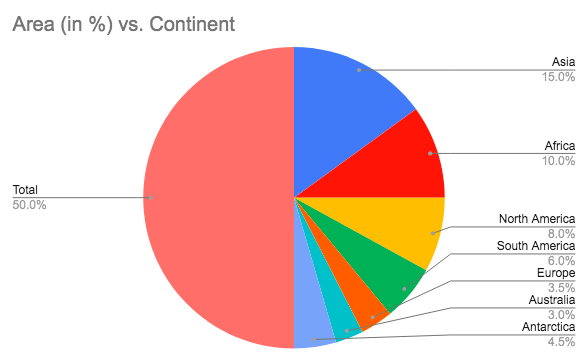Question 2: Draw a histogram for the given frequency distribution.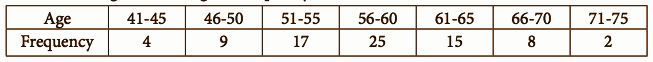Solution:

The given distribution is discontinuous.

Lower boundary = Lower limit – (1 / 2) (gap between the adjacent class interval)

= 41 – (1 / 2) * (1) = 40.5

Upper boundary = Upper limit + (1 / 2) (gap between the adjacent class interval)

= 45 + (1 / 2) (1) = 45.5

The continuous frequency table is as follows:

 Age 40.5 – 45.5 45.5 – 50.5 50.5 – 55.5 55.5 – 60.5 60.5 – 65.5 65.5 – 70.5 70.5 – 75.5 Frequency 4 9 17 25 15 8 2